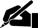# Term Paper: Astronomy

Pages: 3 (870 words)  ·  Bibliography Sources: 3  ·  Level: Master's  ·  Topic: Astronomy  ·  Buy This Paper

Astronomy

Comet Halley has an orbital period of 75 years and when it enters the inner Solar System, it passes within a few AU of the Sun.

Determine the average distance of Comet Halley in AU.

The relation of the orbital period to the semi-major axis is given by the equation P2=a3, where P. is the orbital period and a is the semi-major axis. P is given as 75 and taking the cube root of both sides leaves 752/3=a, or a=17.78. The average distance of Comet Halley (assuming "a few AU" can be interpreted as close to 0) is thus about 18 AU.

Right now, Comet Halley is just about past the orbit of Neptune, which has an average distance from the sun of about 30 AU. Explain how this is possible given the average distance you found from its orbital period.

AU represents the average distance because it is half the major axis measurement, meaning the comet travels twice this linear distance in the completion of its orbit. Comet Halley as a very elliptical orbit if it traverses this distance in this time period, which explains why it can be near Neptune now and back near the sun in about 32.5 years (half its orbital period).

HIPPARCOS (an acronym for HIgh Precision PARallax COllecting Satellite) was a scientific mission of the European Space Agency (ESA), launched in 1989 and operated between 1989 and 1993. It was the first space experiment devoted to astrometry, the accurate measurement of star positions, distances from us, parallaxes, and proper motions.

(a). If the measured parallax shift of star a was 0.1 arcsecs and the parallax shift of star B. was 0.05 arcsecs, which star was farther away from the Earth, why?

The star with the smaller parallax shift, star B, if farther away from the Earth. Distant objects appear to "move" less than nearer objects -- the parallax shift is smaller for distant objects as the Earth moves along its orbit around the Sun.

(b). What is the distance of the farthest star in parsecs?

A parsec is defined as the distance between the Sun and an object with a parallax shift of one arcsec (a 1:1 ratio). Star B. has a parallax shift of 0.05 arcsecs, therefore is at a distance of 1/0.05 parsecs or 20 parsecs.

(c). What is the distance of the farthest star in light-years?

One parsec = approx. 3.26 light years, therefore 20 parsecs = 65.2 light years. Star B. is approx. 65.2ly away.

There… [END OF PREVIEW]

### Ordering Options:

or

2.  Let us write a NEW paper for you!Most popular!

#### Astrophysical Object Phenomenon Thesis…

Cite This Term Paper:

APA Format